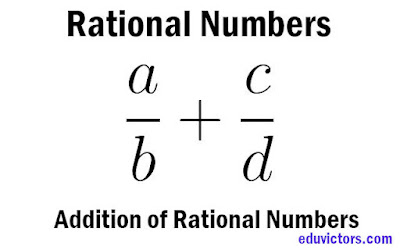# Class 8 Rational Numbers - Question Patterns Related to Addition of Rational Numbers (Solved)

Here, different types of patterns related to addition of rational numbers are given. This will help student understanding the ways to solve these types of questions.

Rational numbers are closed under addition. It means when we add two rational numbers, the resulting number is rational number also.

Q1: Solve $\frac{2}{3} + 0$

$\frac{2}{3} + 0 = \frac{2}{3}$

Note: 0 is called the additive identity for rational numbers. When zero is added to a rational number, the result is the rational number itself.

Q2: Solve $\frac{7}{9} + \frac{1}{9}$

$\frac{7}{9} + \frac{1}{9}$

= $\frac{7+1}{9} = \frac{8}{9}$

Note: In the above question, denominator is same in both rational numbers.

In this case,

$\frac{a}{b} + \frac{c}{b} = \frac{a+c}{b}$

Q3: Solve $\frac{7}{11} + \frac{4}{11}$

$\frac{7}{11} + \frac{4}{11}$

= $\frac{7 + 4}{11}$

= $\frac{11}{11}$ = 1

Q4: Solve $\frac{7}{11} + \frac{-4}{11}$

$\frac{7}{11} + \frac{-4}{11}$

= $\frac{7}{11} - \frac{4}{11}$

= $\frac{7 - 4}{11}$

= $\frac{3}{11}$

Let us see another pattern when denominators are unequal.

Q5: Solve $\frac{2}{3} + \frac{1}{4}$

$\frac{2}{3} + \frac{1}{4}$

= $\frac{2 \times 4 + 1 \times 3}{3 \times 4}$

= $\frac{8 + 3}{12}$

= $\frac{11}{12}$

Note: When there no factor common between denominators, we solve by the following method

$\frac{a}{b} + \frac{c}{d}$

= $\frac{a \times d + b \times c}{b \times d}$

= $\frac{ad + bc}{bd}$

Q6: Solve  $\frac{2}{7} + \frac{3}{14}$

$\frac{2}{7} + \frac{3}{14}$

= $\frac{2 \times 2}{7 \times 2} + \frac{3}{14}$

= $\frac{4}{14} + \frac{3}{14}$

= $\frac{7}{14}$

= $\frac{1}{2}$

Note: In the above example, denominator of the first rational number is multiple of denominator of the second rational number.

Q7: Solve $\frac{-5}{6} + \frac{4}{9}$

$\frac{-5}{6} + \frac{4}{9}$

LCM of 6 and 9 = 3 × 3 × 2 = 18

$\frac{-5}{6} = \frac{-5 \times 3}{6 \times 3} = \frac{-15}{18}$

$\frac{4}{9} = \frac{4 \times 2}{9 \times 2} = \frac{8}{18}$

Thus we have,

= $\frac{-15}{18} + \frac{8}{18}$

= $\frac{-15 + 8}{18}$

= $\frac{-7}{18}$

Note: When there are some common factors between the denominators, we take LCM of denominators.

Q8: Solve $\frac{2}{3} + \frac{1}{6} + \frac{3}{4}$

Answer: LCM of 3, 4 and 6 = 12

$\frac{2}{3} = \frac{2 \times 4}{3 \times 4} = \frac{8}{12}$

$\frac{1}{6} = \frac{1 \times 2}{6 \times 2} = \frac{2}{12}$

$\frac{3}{4} = \frac{3 \times 3}{4 \times 3} = \frac{9}{12}$

$\frac{2}{3} + \frac{1}{6} + \frac{3}{4}$

= $\frac{8}{12} + \frac{2}{12} + \frac{9}{12}$

= $\frac{19}{12}$

Q9: Solve $\frac{2}{7} + 1$

$\frac{2}{7} + 1$

1 = $\frac{7}{7}$

= $\frac{2}{7} + \frac{7}{7}$

= $\frac{9}{7} = 1\frac{2}{7}$

Two rational numbers can be added in any order.

i.e. $\frac{a}{b} + \frac{c}{d} = \frac{c}{d} + \frac{a}{b}$

Q10: Verify $\frac{-2}{3} + \frac{5}{7} = \frac{5}{7} + \frac{-2}{3}$

LHS = $\frac{-2}{3} + \frac{5}{7}$

= $\frac{-2}{3} + \frac{5}{7}$

= $\frac{-2 \times 7 + 3 \times 5}{3 \times 7}$

= $\frac{-14 + 15}{21}$

= $\frac{1}{21}$

RHS = $\frac{5}{7} + \frac{-2}{3}$

= $\frac{5}{7} + \frac{-2}{3}$

= $\frac{5 \times 3 - 2 \times 7}{7 \times 3}$

= $\frac{15-14}{21}$

= $\frac{1}{21}$

Hence LSH = RHS